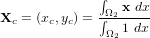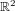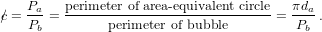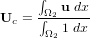## Verification of the results by the picture norm:

To visually verify the results the following approximate bubble shapes can be used. (The raw data for the bubble shapes can be obtained from the Reference data page.)

## Verification of the results by quantitative data:

Since mere visual inspection is not really enough to say anything about the accuracy of the simulations the following benchmark quantities have been defined and computed.

#### Center of Mass

The centroid or center of mass of the bubble is defined by$\begin{displaymath}\mathbf{X}_c= (x_c,y_c)= \frac{\int_{\Omega_2} \mathbf{x}\ dx}{\int_{\Omega_2} 1\ dx}\end{displaymath}$
where Ω2 denotes the region that the bubble occupies.

#### Circularity

The "degree of circularity" in$\mathbb{R}^2$ can be defined as$\begin{displaymath}\slashed{c} = \frac{P_a}{P_b} = \frac{\textrm{perimeter of area-equivalent circle}} {\textrm{perimeter of bubble}} = \frac{\pi d_a}{P_b}\,.\end{displaymath}$

Here, Pa denotes the perimeter or circumference of a circle with diameter da which has an area equal to that of a bubble with perimeter Pb. For a perfectly circular bubble or drop the circularity will be equal to unity and decrease as the bubble is deformed.

#### Rise Velocity

The mean velocity with which a bubble is rising or moving is a particularly interesting quantity since it does not only measure how the interface tracking algorithm behaves but also the quality of the overall solution. The mean bubble velocity is defined as$\begin{displaymath}\mathbf{U}_c = \frac{\int_{\Omega_2} \mathbf{u}\ dx}{\int_{\Omega_2} 1\ dx} \end{displaymath}$
where Ω2 again denotes the region that the bubble occupies.